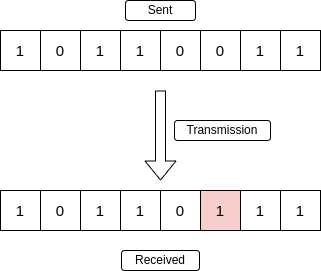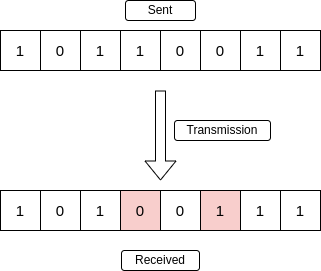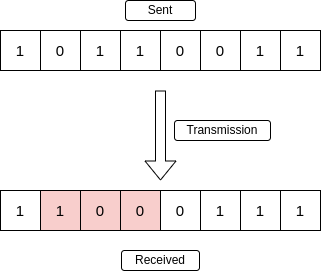GeeksforGeeks App
Open AppBrowser
Continue

# Error Detection in Computer Networks

Error is a condition when the receiver’s information does not match the sender’s information. During transmission, digital signals suffer from noise that can introduce errors in the binary bits traveling from sender to receiver. That means a 0 bit may change to 1 or a 1 bit may change to 0.

Data (Implemented either at the Data link layer or Transport Layer of the OSI Model) may get scrambled by noise or get corrupted whenever a message is transmitted. To prevent such errors, error-detection codes are added as extra data to digital messages. This helps in detecting any errors that may have occurred during message transmission.

## Types of Errors

### Single-Bit Error

A single-bit error refers to a type of data transmission error that occurs when one bit (i.e., a single binary digit) of a transmitted data unit is altered during transmission, resulting in an incorrect or corrupted data unit.Single-Bit Error

### Multiple-Bit Error

A multiple-bit error is an error type that arises when more than one bit in a data transmission is affected. Although multiple-bit errors are relatively rare when compared to single-bit errors, they can still occur, particularly in high-noise or high-interference digital environments.Multiple-Bit Error

### Burst Error

When several consecutive bits are flipped mistakenly in digital transmission, it creates a burst error. This error causes a sequence of consecutive incorrect values.Burst Error

To detect errors, a common technique is to introduce redundancy bits that provide additional information. Various techniques for error detection include::

1. Simple Parity Check
2. Two-dimensional Parity Check
3. Checksum
4. Cyclic Redundancy Check (CRC)

## Error Detection Methods

### Simple Parity Check

Simple-bit parity is a simple error detection method that involves adding an extra bit to a data transmission. It works as:

• 1 is added to the block if it contains an odd number of 1’s, and
• 0 is added if it contains an even number of 1’s

This scheme makes the total number of 1’s even, that is why it is called even parity checking.• Single Parity check is not able to detect even no. of bit error.
• For example, the Data to be transmitted is 101010. Codeword transmitted to the receiver is 1010101 (we have used even parity).
Let’s assume that during transmission, two of the bits of code word flipped to 1111101.
On receiving the code word, the receiver finds the no. of ones to be even and hence no error, which is a wrong assumption.

### Two-dimensional Parity Check

Two-dimensional Parity check bits are calculated for each row, which is equivalent to a simple parity check bit. Parity check bits are also calculated for all columns, then both are sent along with the data. At the receiving end, these are compared with the parity bits calculated on the received data.

### Checksum

Checksum error detection is a method used to identify errors in transmitted data. The process involves dividing the data into equally sized segments and using a 1’s complement to calculate the sum of these segments. The calculated sum is then sent along with the data to the receiver. At the receiver’s end, the same process is repeated and if all zeroes are obtained in the sum, it means that the data is correct.

#### Checksum – Operation at Sender’s Side

• Firstly, the data is divided into k segments each of m bits.
• On the sender’s end, the segments are added using 1’s complement arithmetic to get the sum. The sum is complemented to get the checksum.
• The checksum segment is sent along with the data segments.

#### Checksum – Operation at Receiver’s Side

• At the receiver’s end, all received segments are added using 1’s complement arithmetic to get the sum. The sum is complemented.
• If the result is zero, the received data is accepted; otherwise discarded.

• If one or more bits of a segment are damaged and the corresponding bit or bits of opposite value in a second segment are also damaged.

### Cyclic Redundancy Check (CRC)

• Unlike the checksum scheme, which is based on addition, CRC is based on binary division.
• In CRC, a sequence of redundant bits, called cyclic redundancy check bits, are appended to the end of the data unit so that the resulting data unit becomes exactly divisible by a second, predetermined binary number.
• At the destination, the incoming data unit is divided by the same number. If at this step there is no remainder, the data unit is assumed to be correct and is therefore accepted.
• A remainder indicates that the data unit has been damaged in transit and therefore must be rejected.

Example: Previous year GATE questions based on error detection: GATE CS 2009 Question 48 GATE CS 2007 Question 68. This article has been contributed by Vikash Kumar. Please write comments if you find anything incorrect, or if you want to share more information about the topic discussed above.

Increased Data Reliability: Error detection ensures that the data transmitted over the network is reliable, accurate, and free from errors. This ensures that the recipient receives the same data that was transmitted by the sender.

Improved Network Performance: Error detection mechanisms can help to identify and isolate network issues that are causing errors. This can help to improve the overall performance of the network and reduce downtime.

Enhanced Data Security: Error detection can also help to ensure that the data transmitted over the network is secure and has not been tampered with.